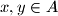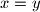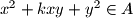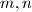### IMO Shortlist 2012 problem N1

Kvaliteta:
Avg: 2,5
Težina:
Avg: 6,0
Call admissible a set$A$ of integers that has the following property:
If$x,y \in A$ (possibly$x=y$) then$x^2+kxy+y^2 \in A$ for every integer$k$.
Determine all pairs$m,n$ of nonzero integers such that the only admissible set containing both$m$ and$n$ is the set of all integers.

Proposed by Warut Suksompong, Thailand
Izvor: Međunarodna matematička olimpijada, shortlist 2012